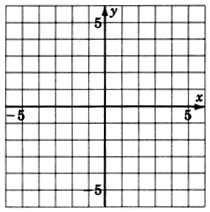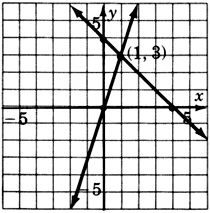# 11.3 Elimination by addition  (Page 2/2)

 Page 2 / 2

## Practice set a

$\left\{\begin{array}{l}x+y=6\\ 2x-y=0\end{array}$

$\left(2,4\right)$

$\left\{\begin{array}{l}x+6y=8\\ -x-2y=0\end{array}$

$\left(-4,2\right)$

## Sample set b

Solve the following systems using the addition method.

Solve $\left\{\begin{array}{rrr}\hfill 6a-5b=14& \hfill & \hfill \left(1\right)\\ \hfill 2a+2b=-10& \hfill & \hfill \left(2\right)\end{array}$

Step 1: The equations are already in the proper form, $ax+by=c.$

Step 2: If we multiply equation (2) by —3, the coefficients of $a$ will be opposites and become 0 upon addition, thus eliminating $a$ .

$\begin{array}{lllll}\left\{\begin{array}{l}6a-5b=14\\ -3\left(2a+2b\right)=-3\left(10\right)\end{array}\hfill & \hfill & \to \hfill & \hfill & \left\{\begin{array}{l}6a-5b=14\\ -6a-6b=30\end{array}\hfill \end{array}$

$\frac{\begin{array}{c}6a-5b=14\\ -6a-6b=30\end{array}}{0-11b=44}$

Step 4:  Solve the equation $-11b=44.$

$-11b=44$
$b=-4$

Step 5:  Substitute $b=-4$ into either of the original equations. We will use equation 2.

$\begin{array}{rrrrr}\hfill 2a+2b& \hfill =& \hfill -10& \hfill & \hfill \\ \hfill 2a+2\left(-4\right)& \hfill =& \hfill -10& \hfill & \hfill \text{Solve\hspace{0.17em}for\hspace{0.17em}}a.\\ \hfill 2a-8& \hfill =& \hfill -10& \hfill & \hfill \\ \hfill 2a& \hfill =& \hfill -2& \hfill & \hfill \\ \hfill a& \hfill =& \hfill -1& \hfill & \hfill \end{array}$

We now have $a=-1$ and $b=-4.$

Step 6:  Substitute $a=-1$ and $b=-4$ into both the original equations for a check.

$\begin{array}{rrrrrrrrrrrr}\hfill \left(1\right)& \hfill & \hfill 6a-5b& \hfill =& \hfill 14& \hfill & \hfill \left(2\right)& \hfill & \hfill 2a+2b& \hfill =& \hfill -10& \hfill \\ \hfill & \hfill & \hfill 6\left(-1\right)-5\left(-4\right)& \hfill =& \hfill 14& \text{Is\hspace{0.17em}this\hspace{0.17em}correct?}\hfill & \hfill & \hfill & \hfill 2\left(-1\right)+2\left(-4\right)& \hfill =& \hfill -10& \text{Is\hspace{0.17em}this\hspace{0.17em}correct?}\hfill \\ \hfill & \hfill & \hfill -6+20& \hfill =& \hfill 14& \text{Is\hspace{0.17em}this\hspace{0.17em}correct?}\hfill & \hfill & \hfill & \hfill -2-8& \hfill =& \hfill -10& \text{Is\hspace{0.17em}this\hspace{0.17em}correct?}\hfill \\ \hfill & \hfill & \hfill 14& \hfill =& \hfill 14& \text{Yes,\hspace{0.17em}this\hspace{0.17em}is\hspace{0.17em}correct}\text{.}\hfill & \hfill & \hfill & \hfill -10& \hfill =& \hfill -10& \text{Yes,\hspace{0.17em}this\hspace{0.17em}is\hspace{0.17em}correct}\text{.}\hfill \end{array}$

Step 7:  The solution is $\left(-1,-4\right).$

Solve  $\left\{\begin{array}{ccc}\begin{array}{l}3x+2y=-4\hfill \\ 4x=5y+10\hfill \end{array}& & \begin{array}{l}\left(1\right)\hfill \\ \left(2\right)\hfill \end{array}\end{array}$

Step 1:  Rewrite the system in the proper form.

$\left\{\begin{array}{ccc}\begin{array}{l}3x+2y=-4\\ 4x-5y=10\end{array}& & \begin{array}{l}\left(1\right)\\ \left(2\right)\end{array}\end{array}$

Step 2:  Since the coefficients of $y$ already have opposite signs, we will eliminate $y$ .
Multiply equation (1) by 5, the coefficient of $y$ in equation 2.
Multiply equation (2) by 2, the coefficient of $y$ in equation 1.

$\begin{array}{rrrrr}\hfill \left\{\begin{array}{l}5\left(3x+2y\right)=5\left(-4\right)\\ 2\left(4x-5y\right)=2\left(10\right)\end{array}& \hfill & \hfill \to & \hfill & \hfill \left\{\begin{array}{l}15x+10y=-20\\ 8x-10y=20\end{array}\end{array}$

$\frac{\begin{array}{c}15x+10y=-20\\ 8x-10y=20\end{array}}{23x+0=0}$

Step 4:  Solve the equation $23x=0$

$23x=0$

$x=0$

Step 5:  Substitute $x=0$ into either of the original equations. We will use equation 1.

$\begin{array}{rrrrr}\hfill 3x+2y& \hfill =& \hfill -4& \hfill & \hfill \\ \hfill 3\left(0\right)+2y& \hfill =& \hfill -4& \hfill & \hfill \text{Solve\hspace{0.17em}for\hspace{0.17em}}y.\\ \hfill 0+2y& \hfill =& \hfill -4& \hfill & \hfill \\ \hfill y& \hfill =& \hfill -2& \hfill & \hfill \end{array}$

We now have $x=0$ and $y=-2.$

Step 6:  Substitution will show that these values check.

Step 7:  The solution is $\left(0,-2\right).$

## Practice set b

Solve each of the following systems using the addition method.

$\left\{\begin{array}{l}3x+y=1\\ 5x+y=3\end{array}$

$\left(1,-2\right)$

$\left\{\begin{array}{l}x+4y=1\\ x-2y=-5\end{array}$

$\left(-3,1\right)$

$\left\{\begin{array}{l}2x+3y=-10\\ -x+2y=-2\end{array}$

$\left(-2,-2\right)$

$\left\{\begin{array}{l}5x-3y=1\\ 8x-6y=4\end{array}$

$\left(-1,-2\right)$

$\left\{\begin{array}{l}3x-5y=9\\ 4x+8y=12\end{array}$

$\left(3,0\right)$

## Addition and parallel or coincident lines

When the lines of a system are parallel or coincident, the method of elimination produces results identical to that of the method of elimination by substitution.

If computations eliminate all variables and produce a contradiction, the two lines of the system are parallel and the system is called inconsistent.

If computations eliminate all variables and produce an identity, the two lines of the system are coincident and the system is called dependent.

## Sample set c

Solve $\left\{\begin{array}{rrr}\hfill 2x-y=1& \hfill & \hfill \left(1\right)\\ \hfill 4x-2y=4& \hfill & \hfill \left(2\right)\end{array}$

Step 1: The equations are in the proper form.

Step 2: We can eliminate $x$ by multiplying equation (1) by –2.

$\begin{array}{rrrrr}\hfill \left\{\begin{array}{c}-2\left(2x-y\right)=-2\left(1\right)\\ 4x-2y=4\end{array}& \hfill & \hfill \to & \hfill & \hfill \left\{\begin{array}{c}-4x+2y=-2\\ 4x-2y=4\end{array}\end{array}$

$\frac{\begin{array}{c}-4x+2y=-2\\ 4x-2y=4\end{array}}{\begin{array}{c}0+0=2\\ 0=2\end{array}}$

This is false and is therefore a contradiction. The lines of this system are parallel.  This system is inconsistent.

Solve  $\left\{\begin{array}{rrr}\hfill 4x+8y=8& \hfill & \hfill \left(1\right)\\ \hfill 3x+6y=6& \hfill & \hfill \left(2\right)\end{array}$

Step 1:  The equations are in the proper form.

Step 2:  We can eliminate $x$ by multiplying equation (1) by –3 and equation (2) by 4.

$\begin{array}{rrrrr}\hfill \left\{\begin{array}{c}-3\left(4x+8y\right)=-3\left(8\right)\\ 4\left(3x+6y\right)=4\left(6\right)\end{array}& \hfill & \hfill \to & \hfill & \hfill \left\{\begin{array}{c}-12x-24y=-24\\ 12x+24y=24\end{array}\end{array}$

$\frac{\begin{array}{c}-12x-24y=-24\\ 12x+24y=24\end{array}}{\begin{array}{c}0+0=0\\ 0=0\end{array}}$

This is true and is an identity. The lines of this system are coincident.

This system is dependent.

## Practice set c

Solve each of the following systems using the addition method.

$\left\{\begin{array}{c}-x+2y=6\\ -6x+12y=1\end{array}$

inconsistent

$\left\{\begin{array}{c}4x-28y=-4\\ x-7y=-1\end{array}$

dependent

## Exercises

For the following problems, solve the systems using elimination by addition.

$\left\{\begin{array}{c}x+y=11\\ x-y=-1\end{array}$

$\left(5,6\right)$

$\left\{\begin{array}{c}x+3y=13\\ x-3y=-11\end{array}$

$\left\{\begin{array}{c}3x-5y=-4\\ -4x+5y=2\end{array}$

$\left(2,2\right)$

$\left\{\begin{array}{c}2x-7y=1\\ 5x+7y=-22\end{array}$

$\left\{\begin{array}{c}-3x+4y=-24\\ 3x-7y=42\end{array}$

$\left(0,-6\right)$

$\left\{\begin{array}{c}8x+5y=3\\ 9x-5y=-71\end{array}$

$\left\{\begin{array}{c}-x+2y=-6\\ x+3y=-4\end{array}$

$\left(2,-2\right)$

$\left\{\begin{array}{c}4x+y=0\\ 3x+y=0\end{array}$

$\left\{\begin{array}{c}x+y=-4\\ -x-y=4\end{array}$

dependent

$\left\{\begin{array}{c}-2x-3y=-6\\ 2x+3y=6\end{array}$

$\left\{\begin{array}{c}3x+4y=7\\ x+5y=6\end{array}$

$\left(1,1\right)$

$\left\{\begin{array}{c}4x-2y=2\\ 7x+4y=26\end{array}$

$\left\{\begin{array}{c}3x+y=-4\\ 5x-2y=-14\end{array}$

$\left(-2,2\right)$

$\left\{\begin{array}{c}5x-3y=20\\ -x+6y=-4\end{array}$

$\left\{\begin{array}{c}6x+2y=-18\\ -x+5y=19\end{array}$

$\left(-4,3\right)$

$\left\{\begin{array}{c}x-11y=17\\ 2x-22y=4\end{array}$

$\left\{\begin{array}{c}-2x+3y=20\\ -3x+2y=15\end{array}$

$\left(-1,6\right)$

$\left\{\begin{array}{c}-5x+2y=-4\\ -3x-5y=10\end{array}$

$\left\{\begin{array}{c}-3x-4y=2\\ -9x-12y=6\end{array}$

dependent

$\left\{\begin{array}{c}3x-5y=28\\ -4x-2y=-20\end{array}$

$\left\{\begin{array}{c}6x-3y=3\\ 10x-7y=3\end{array}$

$\left(1,\text{\hspace{0.17em}}1\right)$

$\left\{\begin{array}{c}-4x+12y=0\\ -8x+16y=0\end{array}$

$\left\{\begin{array}{c}3x+y=-1\\ 12x+4y=6\end{array}$

inconsistent

$\left\{\begin{array}{c}8x+5y=-23\\ -3x-3y=12\end{array}$

$\left\{\begin{array}{c}2x+8y=10\\ 3x+12y=15\end{array}$

dependent

$\left\{\begin{array}{c}4x+6y=8\\ 6x+8y=12\end{array}$

$\left\{\begin{array}{c}10x+2y=2\\ -15x-3y=3\end{array}$

inconsistent

$\left\{\begin{array}{c}x+\frac{3}{4}y=-\frac{1}{2}\\ \frac{3}{5}x+y=-\frac{7}{5}\end{array}$

$\left\{\begin{array}{c}x+\frac{1}{3}y=\frac{4}{3}\\ -x+\frac{1}{6}y=\frac{2}{3}\end{array}$

$\left(0,4\right)$

$\left\{\begin{array}{c}8x-3y=25\\ 4x-5y=-5\end{array}$

$\left\{\begin{array}{c}-10x-4y=72\\ 9x+5y=39\end{array}$

$\left(-\frac{258}{7},\frac{519}{7}\right)$

$\left\{\begin{array}{c}12x+16y=-36\\ -10x+12y=30\end{array}$

$\left\{\begin{array}{c}25x-32y=14\\ -50x+64y=-28\end{array}$

dependent

## Exercises for review

( [link] ) Simplify and write ${\left(2{x}^{-3}{y}^{4}\right)}^{5}{\left(2x{y}^{-6}\right)}^{-5}$ so that only positive exponents appear.

( [link] ) Simplify $\sqrt{8}+3\sqrt{50}.$

$17\sqrt{2}$

( [link] ) Solve the radical equation $\sqrt{2x+3}+5=8.$

( [link] ) Solve by graphing $\left\{\begin{array}{c}x+y=4\\ 3x-y=0\end{array}$$\left(1,3\right)$( [link] ) Solve using the substitution method: $\left\{\begin{array}{c}3x-4y=-11\\ 5x+y=-3\end{array}$

how can chip be made from sand
are nano particles real
yeah
Joseph
Hello, if I study Physics teacher in bachelor, can I study Nanotechnology in master?
no can't
Lohitha
where we get a research paper on Nano chemistry....?
nanopartical of organic/inorganic / physical chemistry , pdf / thesis / review
Ali
what are the products of Nano chemistry?
There are lots of products of nano chemistry... Like nano coatings.....carbon fiber.. And lots of others..
learn
Even nanotechnology is pretty much all about chemistry... Its the chemistry on quantum or atomic level
learn
da
no nanotechnology is also a part of physics and maths it requires angle formulas and some pressure regarding concepts
Bhagvanji
hey
Giriraj
Preparation and Applications of Nanomaterial for Drug Delivery
revolt
da
Application of nanotechnology in medicine
has a lot of application modern world
Kamaluddeen
yes
narayan
what is variations in raman spectra for nanomaterials
ya I also want to know the raman spectra
Bhagvanji
I only see partial conversation and what's the question here!
what about nanotechnology for water purification
please someone correct me if I'm wrong but I think one can use nanoparticles, specially silver nanoparticles for water treatment.
Damian
yes that's correct
Professor
I think
Professor
Nasa has use it in the 60's, copper as water purification in the moon travel.
Alexandre
nanocopper obvius
Alexandre
what is the stm
is there industrial application of fullrenes. What is the method to prepare fullrene on large scale.?
Rafiq
industrial application...? mmm I think on the medical side as drug carrier, but you should go deeper on your research, I may be wrong
Damian
How we are making nano material?
what is a peer
What is meant by 'nano scale'?
What is STMs full form?
LITNING
scanning tunneling microscope
Sahil
how nano science is used for hydrophobicity
Santosh
Do u think that Graphene and Fullrene fiber can be used to make Air Plane body structure the lightest and strongest. Rafiq
Rafiq
what is differents between GO and RGO?
Mahi
what is simplest way to understand the applications of nano robots used to detect the cancer affected cell of human body.? How this robot is carried to required site of body cell.? what will be the carrier material and how can be detected that correct delivery of drug is done Rafiq
Rafiq
if virus is killing to make ARTIFICIAL DNA OF GRAPHENE FOR KILLED THE VIRUS .THIS IS OUR ASSUMPTION
Anam
analytical skills graphene is prepared to kill any type viruses .
Anam
Any one who tell me about Preparation and application of Nanomaterial for drug Delivery
Hafiz
what is Nano technology ?
write examples of Nano molecule?
Bob
The nanotechnology is as new science, to scale nanometric
brayan
nanotechnology is the study, desing, synthesis, manipulation and application of materials and functional systems through control of matter at nanoscale
Damian
how did you get the value of 2000N.What calculations are needed to arrive at it
Privacy Information Security Software Version 1.1a
Good
Please keep in mind that it's not allowed to promote any social groups (whatsapp, facebook, etc...), exchange phone numbers, email addresses or ask for personal information on QuizOver's platform.

#### Get Jobilize Job Search Mobile App in your pocket Now!ByByBy Brooke DelaneyBy Jonathan LongBy Brooke DelaneyBy Jonathan LongBy OpenStaxByBy Rebecca ButterfieldByBy Lakeima RobertsBy OpenStax